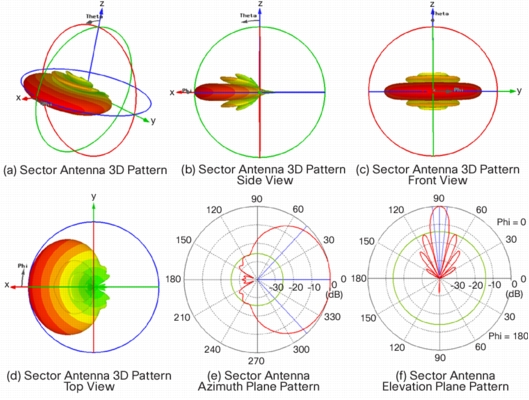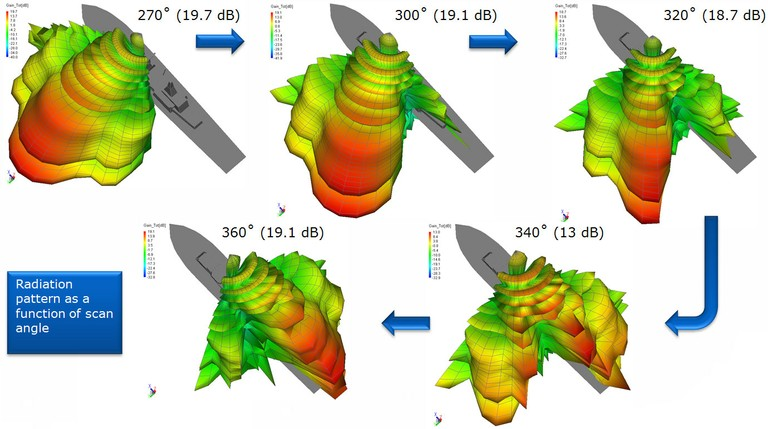# Fundamentals of a Rectangular Array – Mathematical Model and Code

###### Background

In the previous few posts we discussed the fundamentals of Uniform Linear Arrays (ULAs), Beamforming, Multiuser Detection and Massive MIMO (, , , ). Now we turn our attention to more complicated array structures such as rectangular, triangular and circular. We still assume each element of the array to have an isotropic or omni-directional (in the plane of the array) radiation pattern. The mathematical models for more complicated radiation patterns are an extension of  the what is developed here.

###### Square and Rectangular Arrays

In this post we consider a square array which is a special case of rectangular array.  We build up from the most basic case of a 2 x 2 array and derive the equation of the resultant signal, which is simply the summation of the individual signals received at the four array elements. Later on we give the MATLAB code which can be used to plot the radiation pattern of any size rectangular array.

###### Derivation of the Array Pattern

Lets consider a plane wave impinging upon a 2 x 2 receive array. The source is considered to be in the far field of the receive array making the plane wave assumption to be realistic. The equations for the received signal at the four array elements are given below. Please note that since the combined signal only depends upon the relative phase of the four components we assume the phase at the wavefront (red line in the figure below) to be zero. Also note that we have assumed that dx=dy=d.

##### r4=e-j2πd(cos(θ)+sin(θ))/λ

The resultant signal can then be written as:

##### rt=r1+r2+r3+r4

In general for an N x M array the resultant signal can be written as:

##### rt=∑∑ e-j2πd(ncos(θ)+msin(θ))/λ

When the array is not square i.e. the separation along the x and y axes is not the same we have:

##### rt=∑∑ e-j2π(ndxcos(θ)+mdysin(θ))/λ

The range of n and m is 0 to N-1 and 0 to M-1 respectively.

###### MATLAB Code for a Rectangular Array
```%%%%%%%%%%%%%%%%%%%%%%%%%%%%%%%%%%%%
% SIMPLE RECTANGULAR ARRRAY
% WITH N x M ELEMENTS
%%%%%%%%%%%%%%%%%%%%%%%%%%%%%%%%%%%%
clear all
close all

f=1e9;
c=3e8;
l=c/f;
dx=l/3;
dy=l/3;
theta=0:pi/1800:2*pi;
N=8;
M=8;

r=0;
for n=1:N
for m=1:M
r=r+exp(-i*2*pi*(dx*(n-1)*cos(theta)+dy*(m-1)*sin(theta))/l);
end
end

polar(theta,abs(r),'bo-')
title ('Gain of a Uniform Linear Array')
```
###### Discussion of Simulation Results

In the above code we have assumed a carrier frequency of 1 GHz which gives us a wavelength of 0.3 meter. The element separation along the x and y axis is assumed to be 0.1 meter (lambda/3). The total number of elements is 8 x 8 = 64. The maximum gain obtained is equal to 8 on the  linear scale or 9 dB on the logarithmic scale. The radiation pattern has four peaks which does not make this array structure to be of much practical use. More complex array structures resulting in more desirable radiation patterns will be discussed in future posts.

###### Note:

Please note that we have simplified the problem significantly by assuming an omnidirectional pattern of the array elements and plotting the composite radiation pattern only in the plane of the array. In reality the array elements do not always have an omnidirectional radiation pattern (one popular antenna that does have an omnidirectional pattern is a dipole) and we have to plot the 3D pattern of the array (or cuts along different planes) to get a better understanding of the characteristics such as Half Power Beamwidth, First Null Beamwidth and Side Lobe Level etc. More on this later.

# E and H Field of a Patch

The Electric and Magnetic Field variations within a patch are sometimes a bit confusing and difficult to visualize. The figure below shows the E and H Field variations within a rectangular patch of length L and width W.

As can be seen the E-field varies along the length of the patch with minimum at the centre and maximum at the edges (maximum positive and maximum negative). The H-field also varies along the length is in a direction perpendicular to the E-field. The H-field is maximum at the center and minimum at the edges. Thus the impedance is zero at the center of the patch (using Z=V/I).

Another point to note is that the E-field does not completely terminate at the edges along the length of the antenna rather it extends at the outer periphery. These field extensions are known as fringing fields and cause the patch to radiate .

# E-field of a Patch Antenna

A Microstrip Patch Antenna or simply a Patch Antenna is a very common antenna type used in cell phones and many other electronic devices. It basically consists of two metallic plates separated by a dielectric layer. The metallic plates are usually made of copper or some other highly conductive material. Another important feature of this antenna is the feeding mechanism, which is also made of a highly conductive material. A Microstrip Patch Antenna fed by a 50 ohm transmission line and a quarterwave transformer is shown below.

The E-field and H-field generated by the Patch Antenna can be calculated by using a simulation tool such as CST Microwave Studio. It is dependent on the length ‘L’ and width ‘W’ of the patch as well as on the wavelength ‘λ’ and the feeding mechanism. Other important parameters are the width of the substrate and the permittivity of the substrate. The E-field can be approximated as.

Part of the above equations is a sinc function which can be calculated by the MATLAB sinc function.

References:

# Some Commonly Used Antenna Terminology

Antenna Pattern

Antenna Pattern or Radiation Pattern is a three dimensional description of how the antenna radiates energy in to the space around it. All practical antennas are directional i.e. they radiate more energy in certain directions and lesser energy in other directions. Although the Radiation Pattern is a three dimensional quantity it can be described in two perpendicular planes known as the principal planes. Usually one of these planes is horizontal (azimuth plane) and the other is vertical (elevation plane).

Gain

As discussed previously antennas do not radiate uniformly in all directions. Antenna Gain is the ratio of the power transmitted in a certain direction to the power transmitted in that direction by an isotropic source. An isotropic source is an imaginary source which radiates power uniformly in all directions. The Antenna Gain is usually given in units of decibels and is given as dBi (dB above an isotropic source). The Antenna Gain may also be given with reference to a Dipole Antenna and this is labelled as dBd. When no direction is specified Antenna Gain refers to the maximum Gain.

3-dB Beamwidth

The 3-dB Beamwidth is the angle between two points on the main lobe of the antenna that are 3dB down from the maximum value. A smaller Beamwidth means that an antenna is more directional and a higher Beamwidth means that the antenna is less directional. The 3-dB Beamwidth is usually defined in the two principal planes i.e. the azimuth plane and elevation plane.

Front-to-back ratio

The front to back ration (F/B) is the ratio of the Gain in the forward direction to the Gain in the backward direction within a principal plane (azimuth or elevation). It is an important metric in certain scenarios where radiation towards the back of the antenna is undesirable e.g. as in 120 degree sectored antennas used in cellular networks. The ratio is usually given on a logarithmic scale and labelled as dB.

Polarization

The polarization of an antenna is a somewhat difficult concept to comprehend. The polarization of an antenna describes the orientation of the E-field that is generated by the antenna. Usually the orientation is horizontal or vertical but it could also be circular for the case where the E-field rotates (clockwise or counter clockwise) as it travels. If a transmit antenna is vertically polarized then the receive antenna should also be vertically polarized to achieve maximum power transfer through the medium.

VSWR

The voltage standing wave ratio (VSWR) is defined as the ratio of the maximum voltage to the minimum voltage in a standing wave pattern. A ratio of 1:1 means that all power was transferred to the antenna and there are no reflections. Typically RF components have 50 or 75 ohms impedance and antennas must have the same impedance to achieve maximum power transfer.

VSWR bandwidth

The VSWR bandwidth is defined as the frequency range over which an antenna has a specified VSWR. Often, the 2:1 VSWR bandwidth is specified, but 1.5:1 is also common.

Omnidirectional antenna

An omnidirectional antenna is an antenna that has a non-directional pattern (circular pattern) in a given plane with a directional pattern in any orthogonal plane. Examples of omnidirectional antennas are dipoles and collinear antennas.

Reference:
 Cisco Aironet Antennas and Accessories, Antenna Patterns and Their Meaning.

# Some Common Antenna Radiation Patterns

A Radiation Pattern is a 3 dimensional description of how an antenna radiates power in the surrounding space. This pattern is usually measured at a sufficient distance from the antenna known as the far-field. In simple words it is the power radiated in a certain direction with reference to an omni-directional antenna (a theoretical antenna that radiates   equally in all the directions). Given below are the radiation patterns for some common antenna types.Radar Antenna Radiation Patterns